Determinants and the Order of Matrix Multiplication

Showing that the determinant of a product of matrices is the product of determinants

Creating a symbolic matrix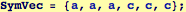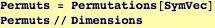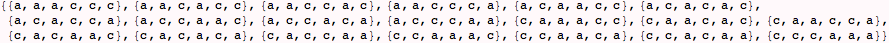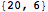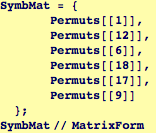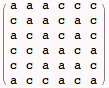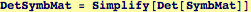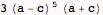Creating a matrix of random  rational numbers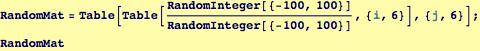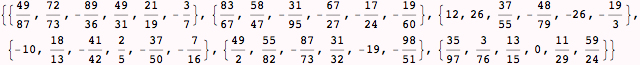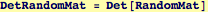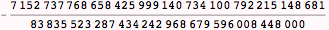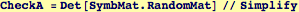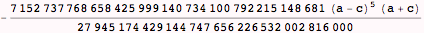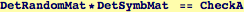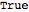Does the determinant of a product depend on the order of multiplication?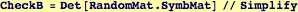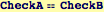However, the product of two matrices depends on which matrix is on the left and which is on the right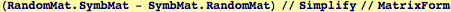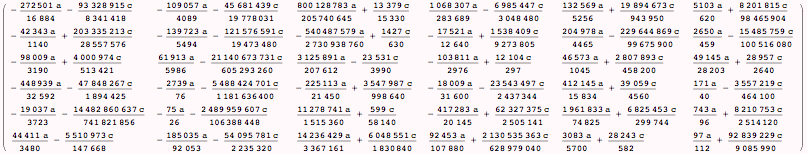Created by Wolfram Mathematica 6.0  (06 September 2007)